# RD Sharma Solutions for Class 8 Maths Chapter 4 - Cubes and Cube Roots Exercise 4.4

Exercise 4.4 of RD Sharma Class 8 Maths Chapter 4 Cubes and Cube Roots, we shall discuss problems based on the cube root of a negative integral perfect cube, cube root of the product of integers, cube root of a rational number. Download free pdf of RD Sharma Class 8 Maths from the links provided below. Students can refer to RD Sharma Class 8 Solutions which are designed specially by our expert team, to provide complete and accurate solutions for each and every question mentioned in the book. This, in turn, yields good results in their exams, when practised on a regular basis.

## Download PDF of RD Sharma Solutions for Class 8 Maths Exercise 4.4 Chapter 4 Cubes and Cube Roots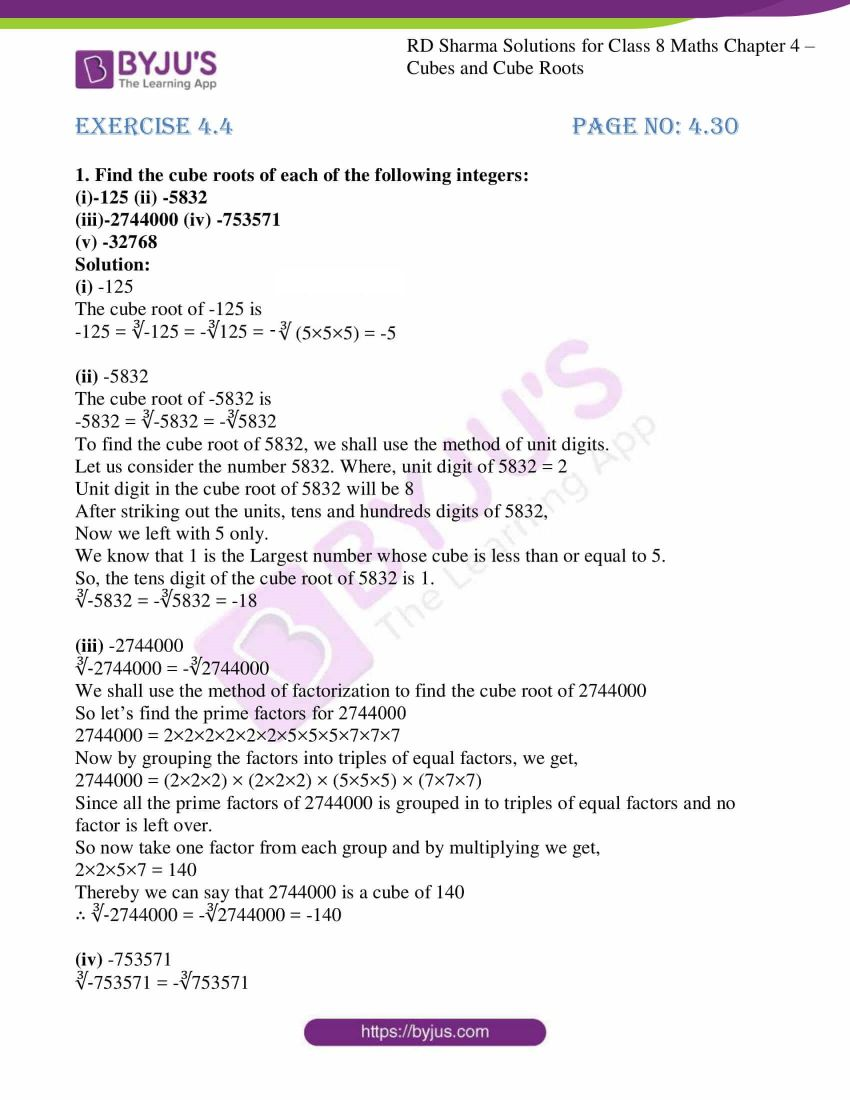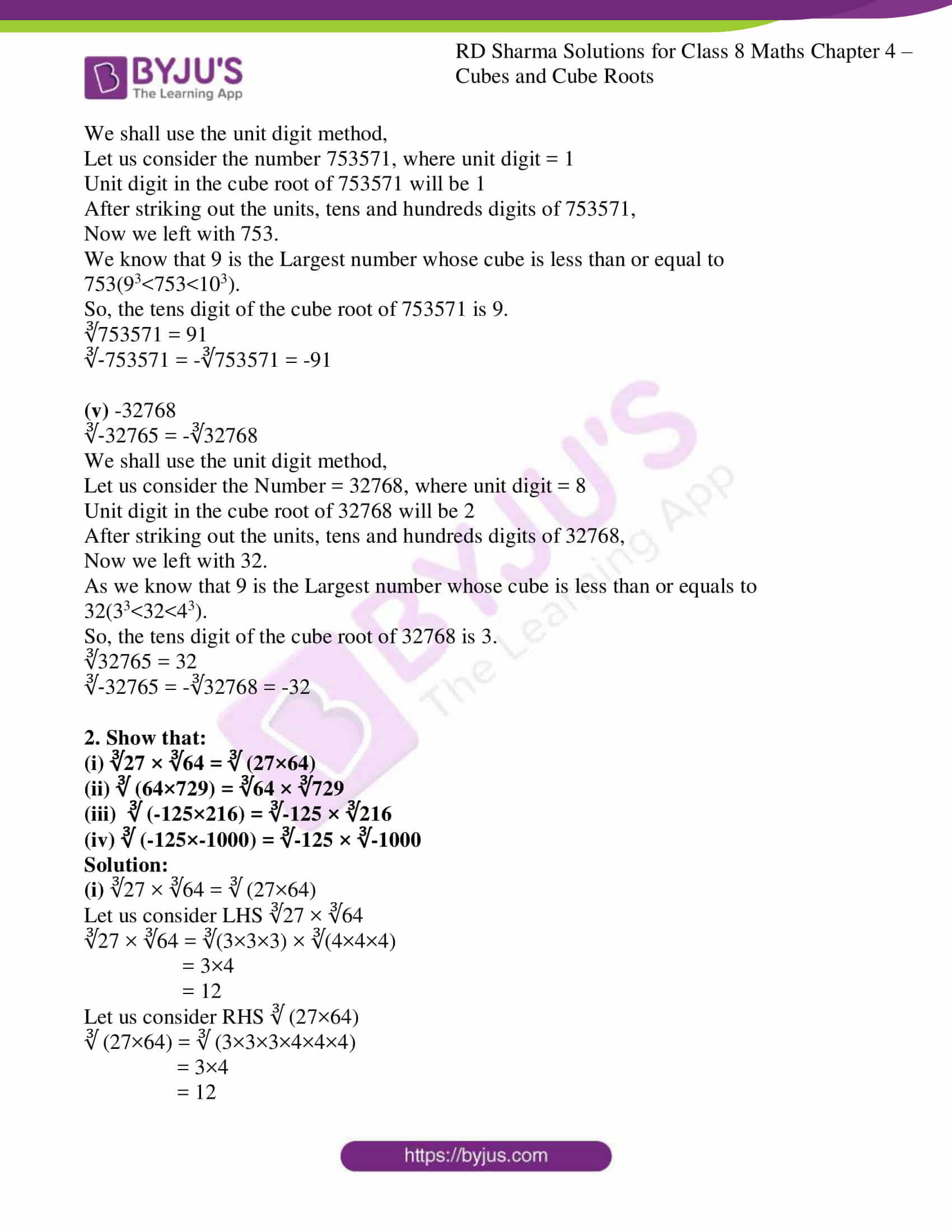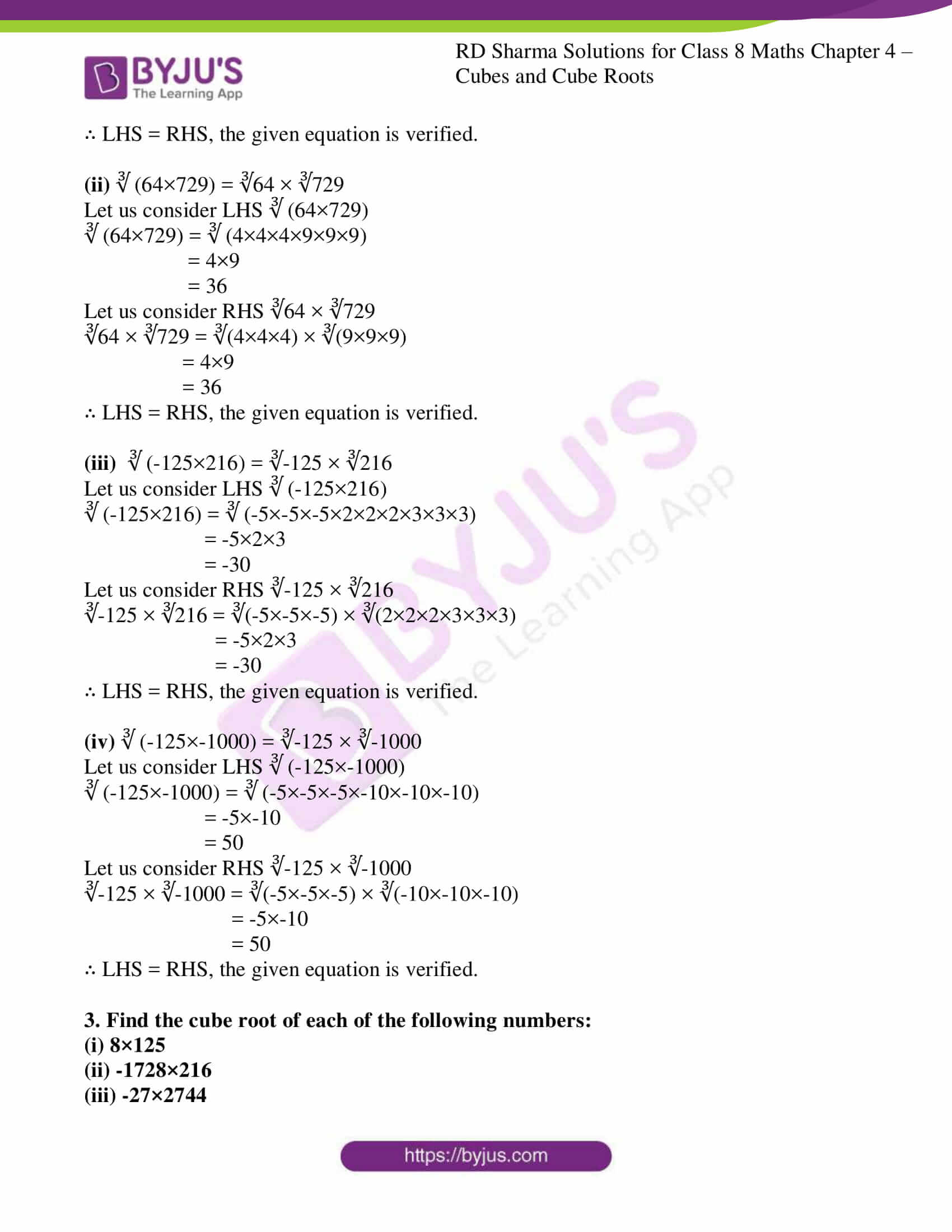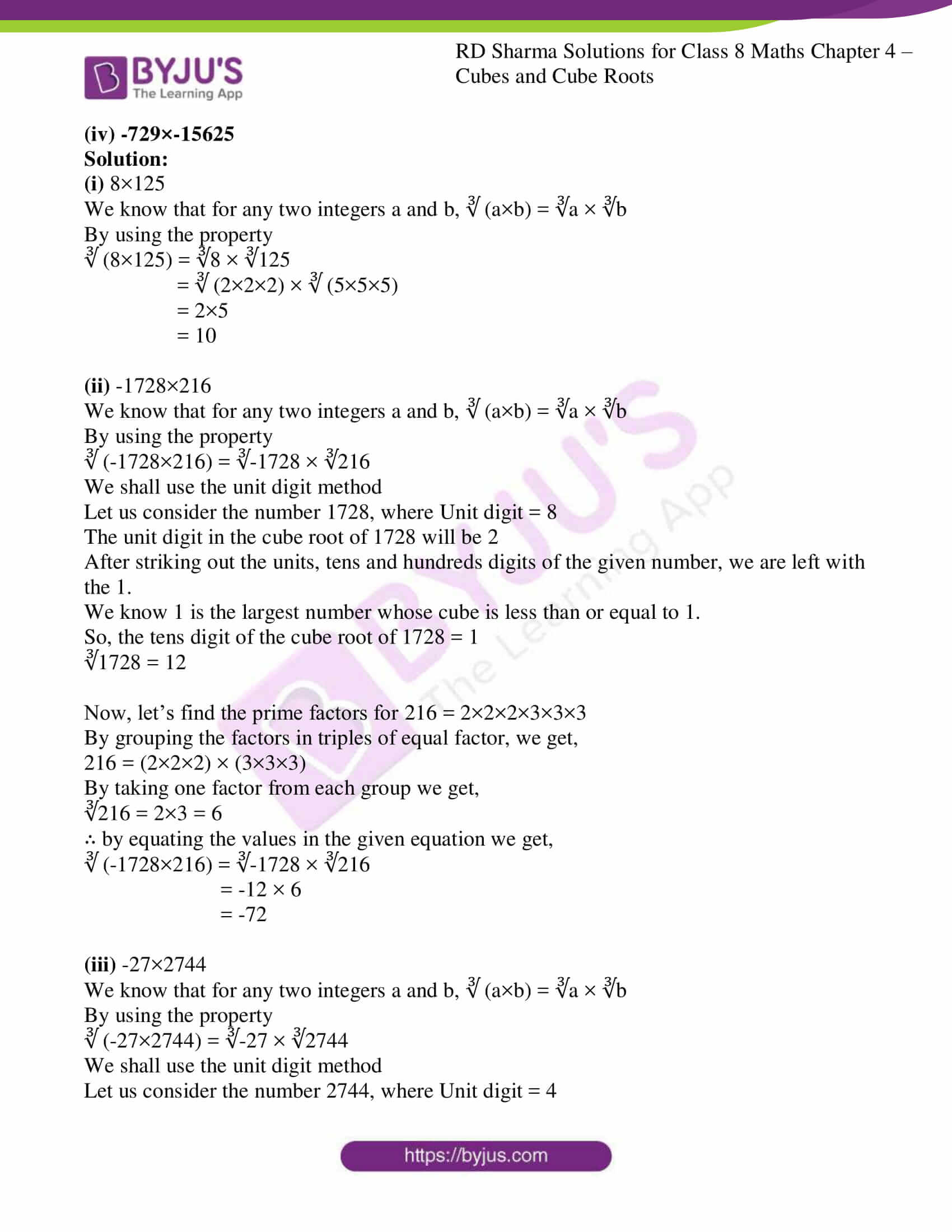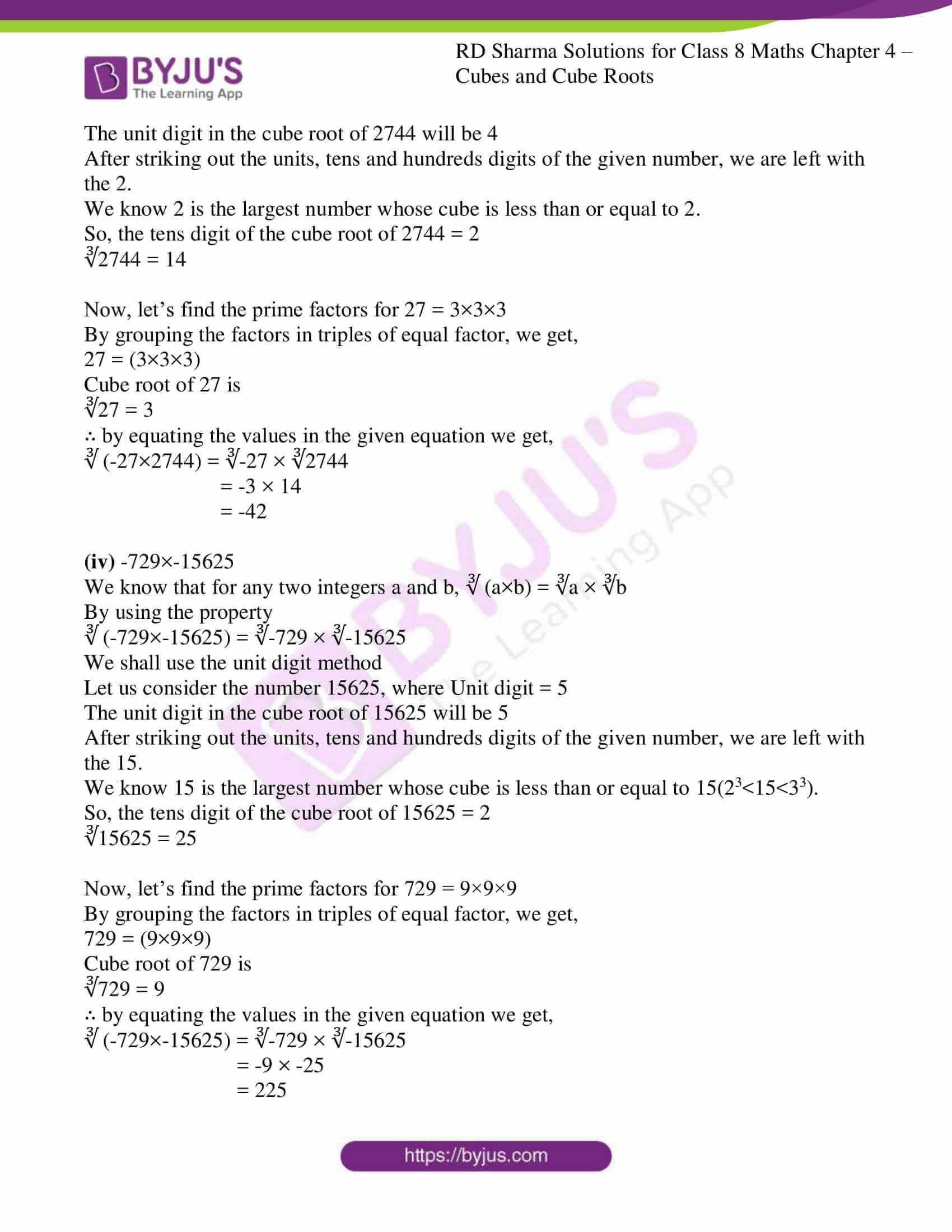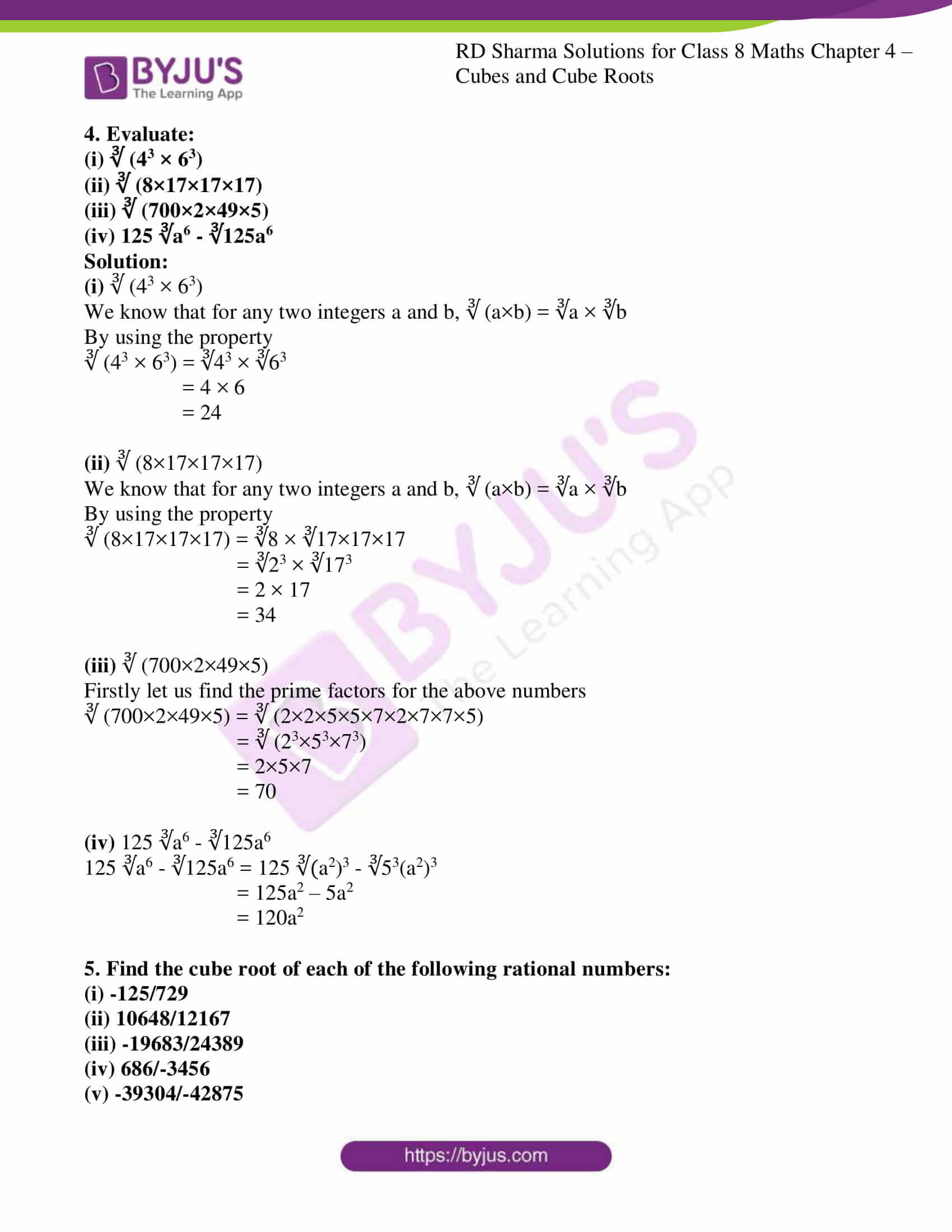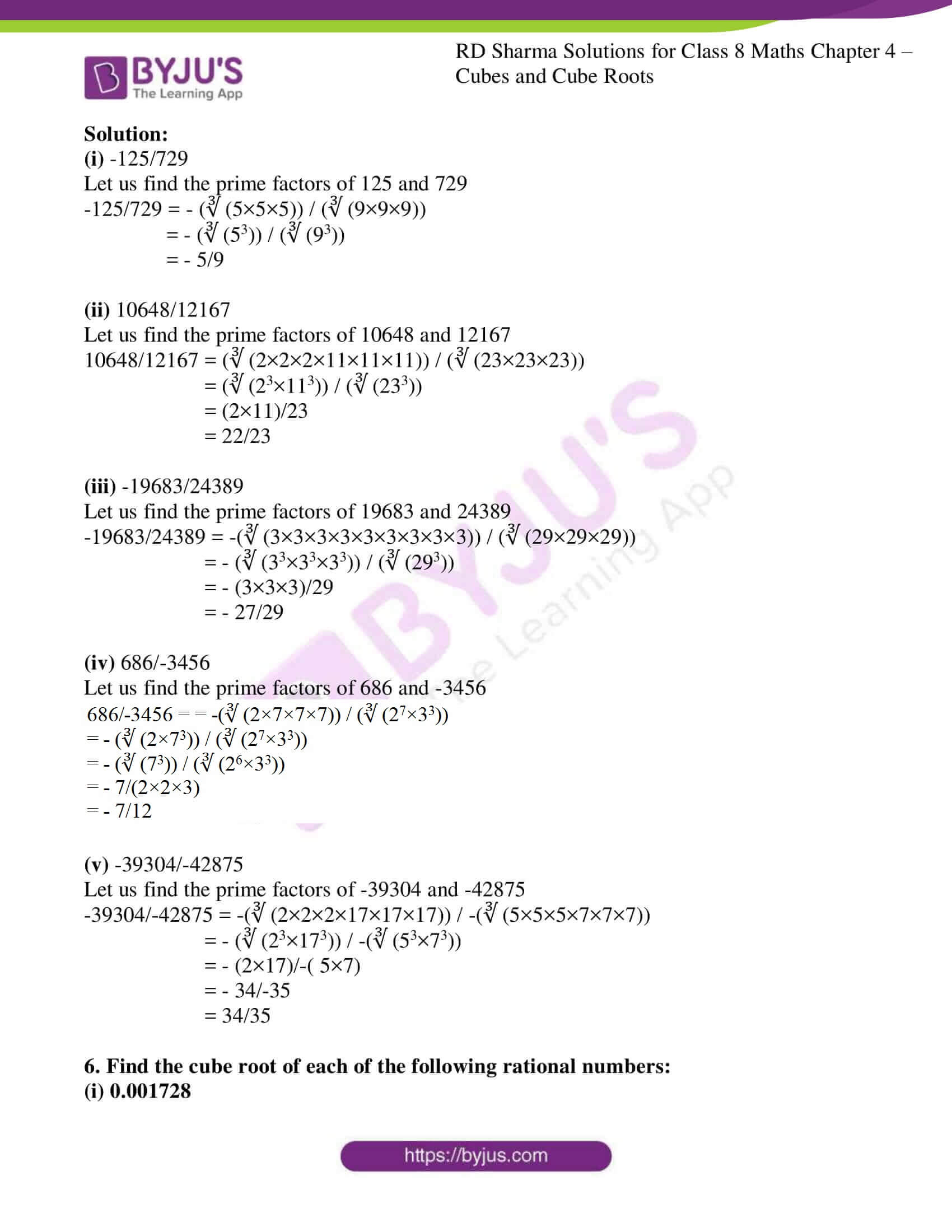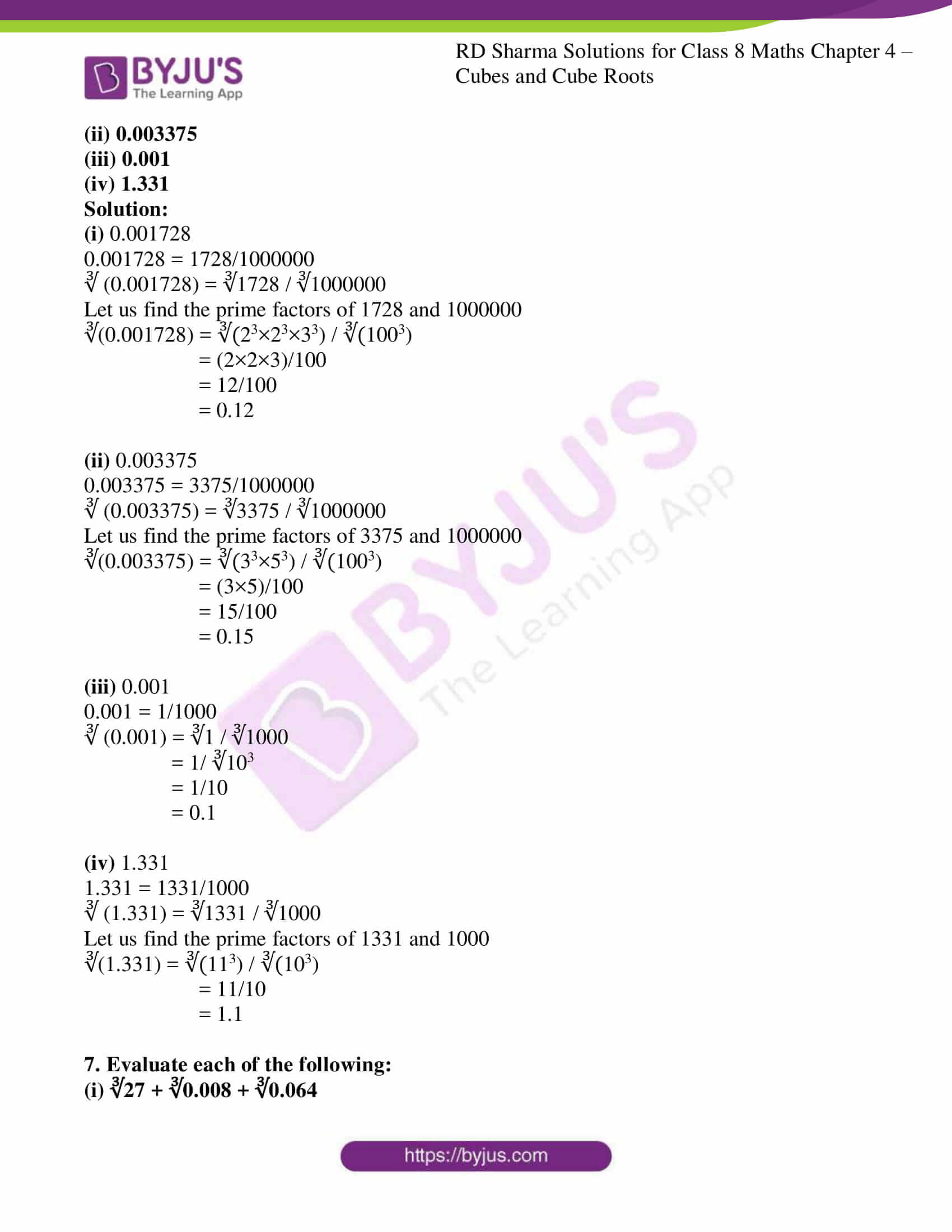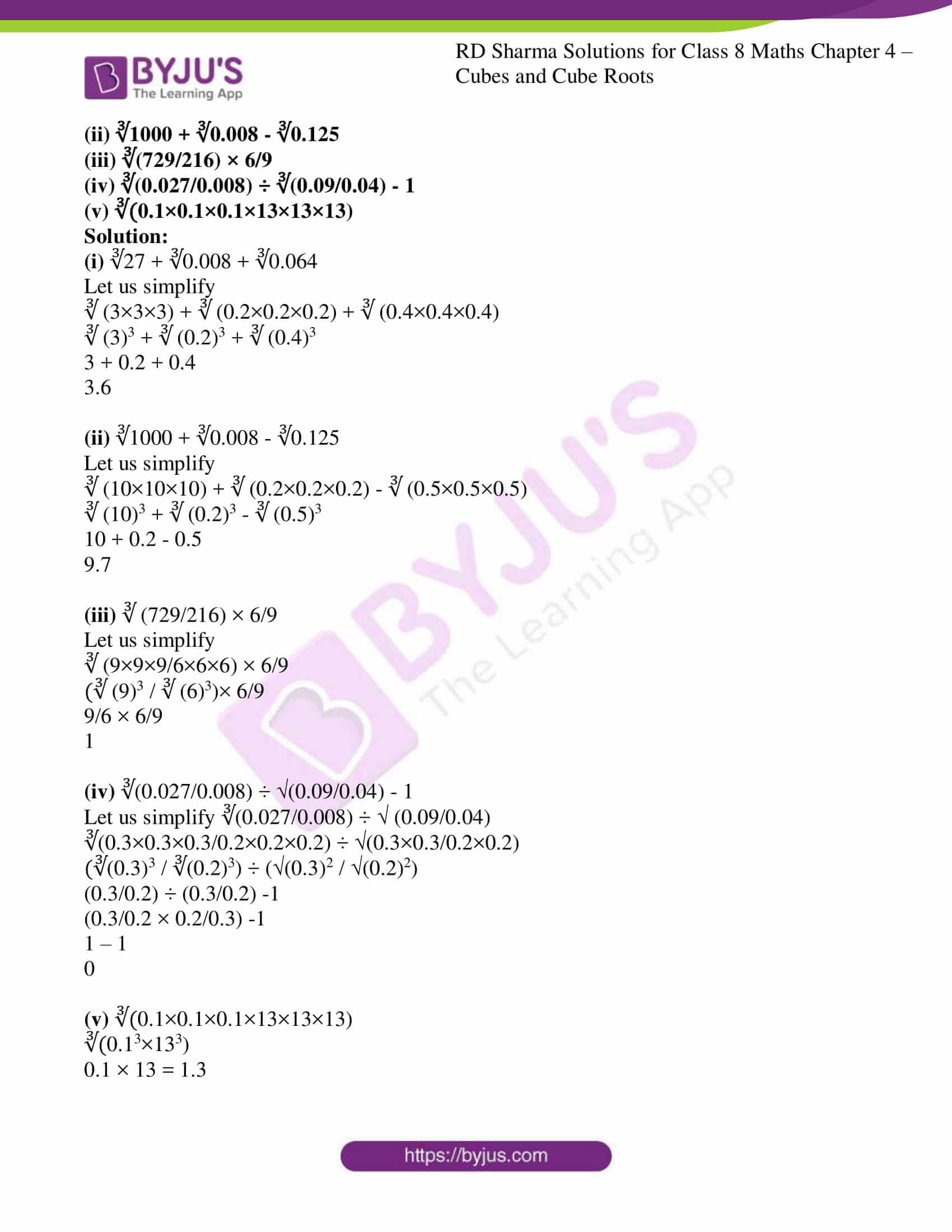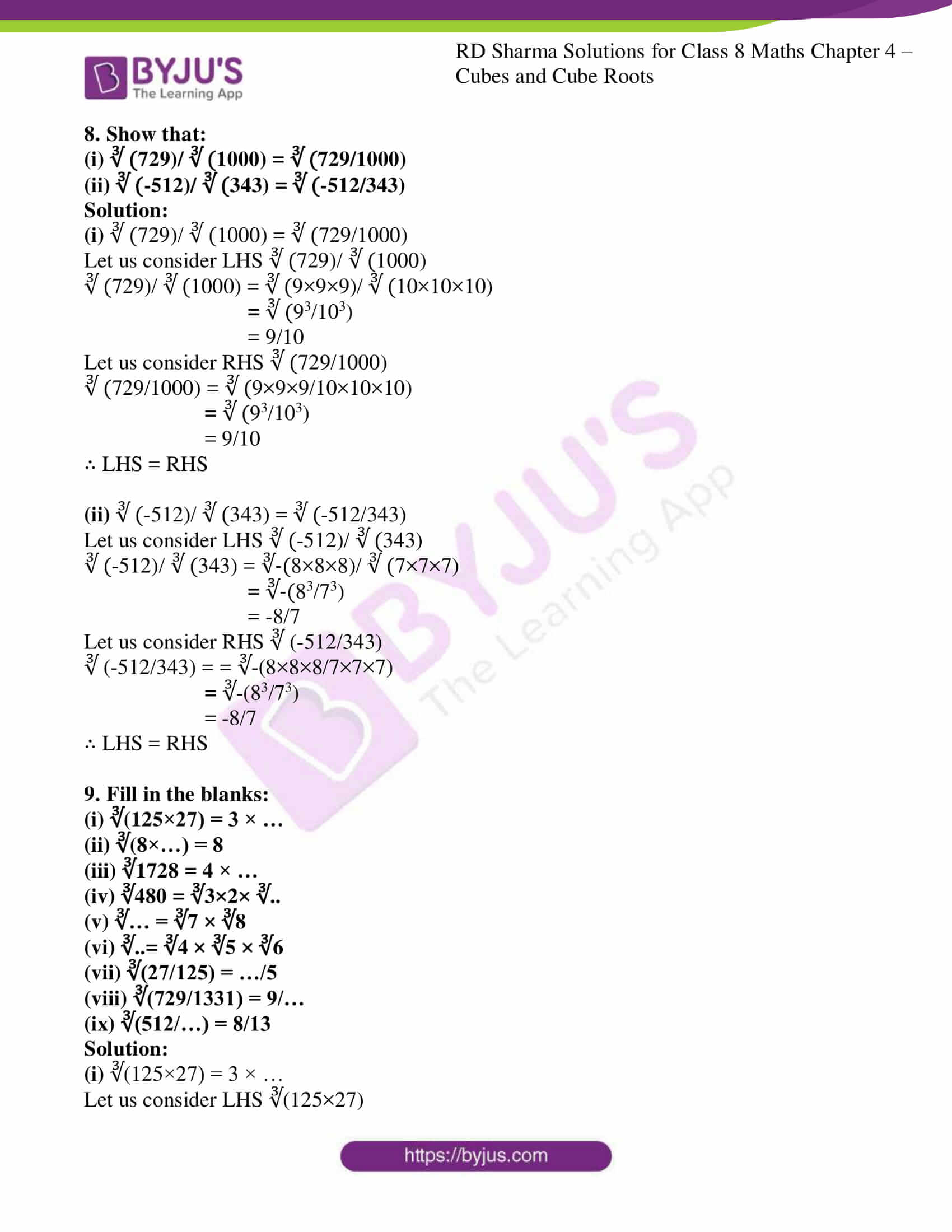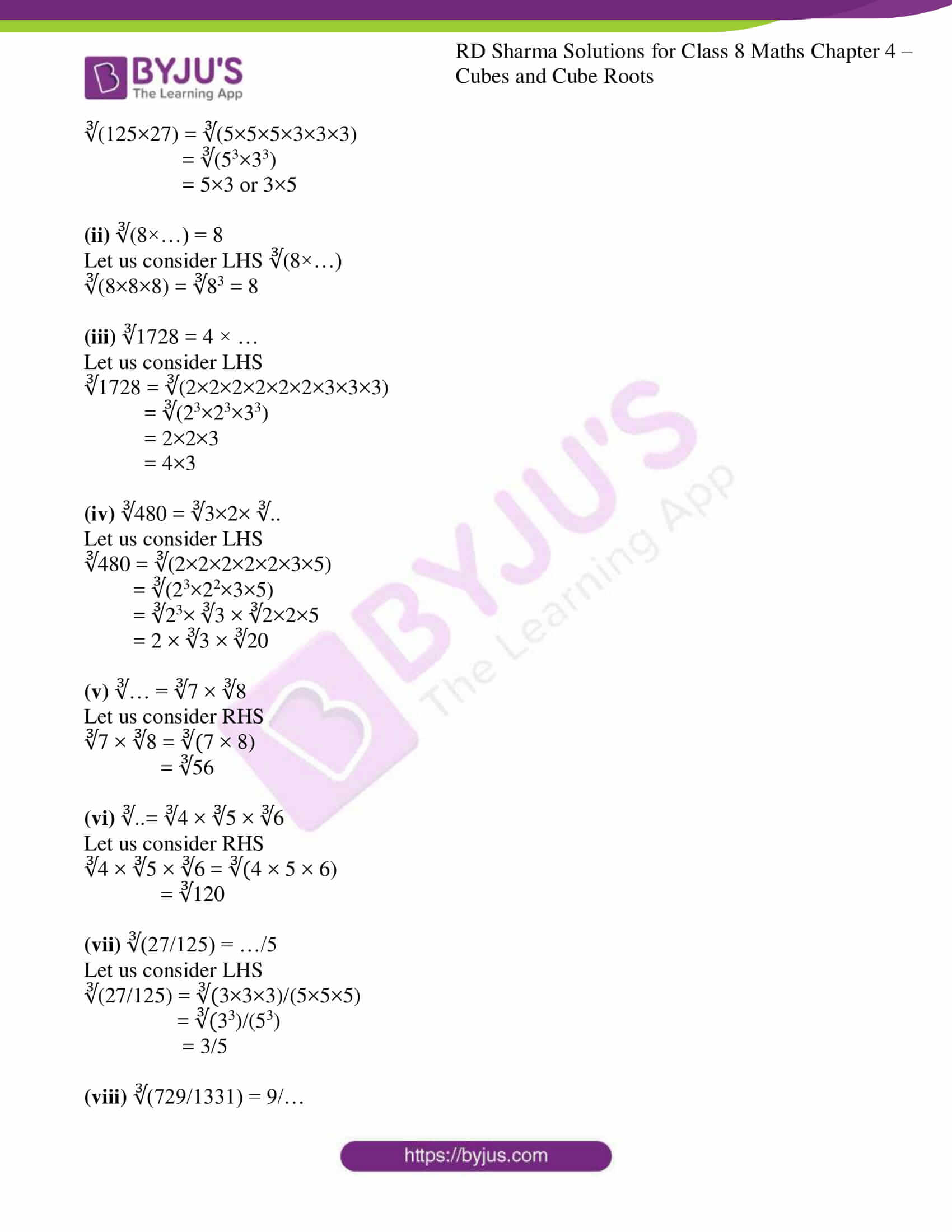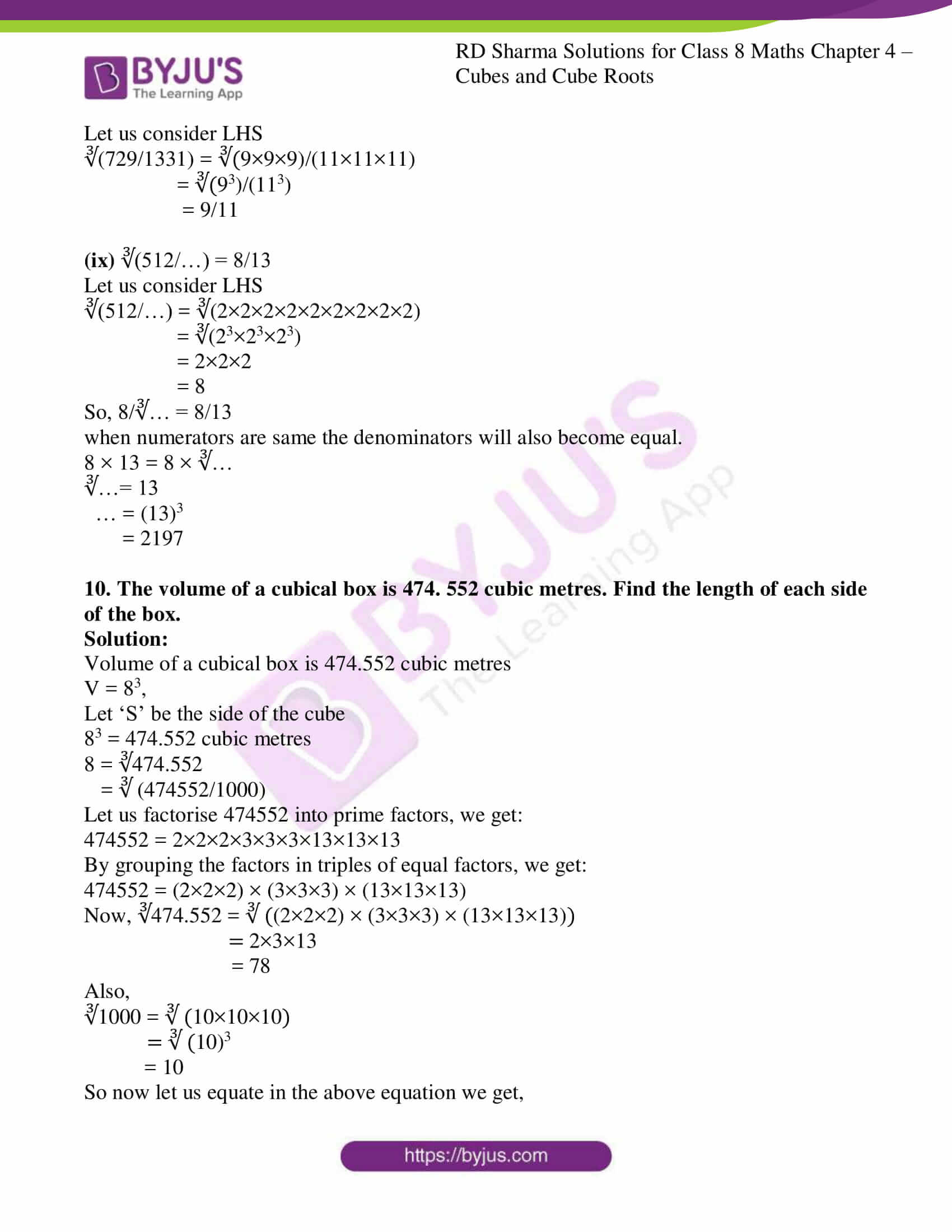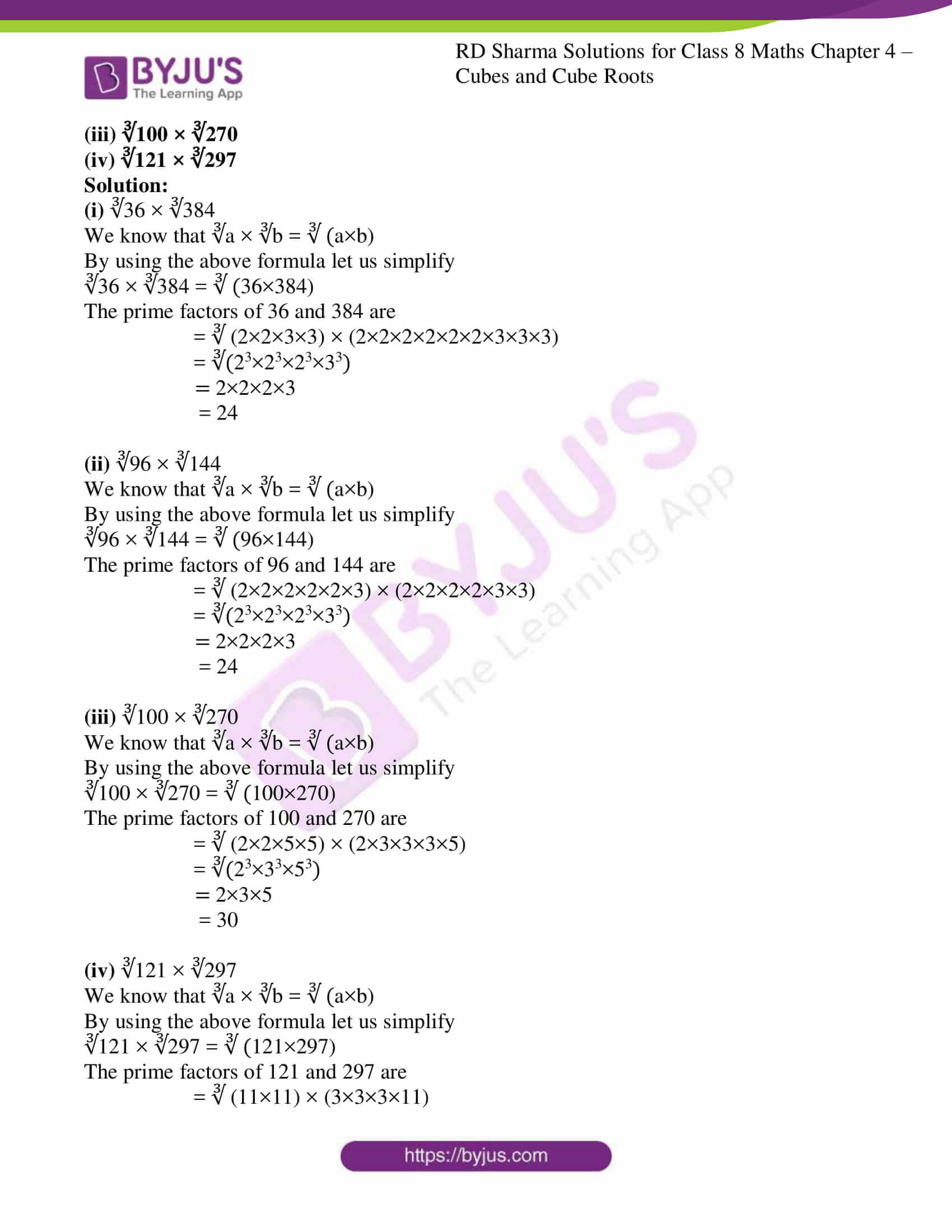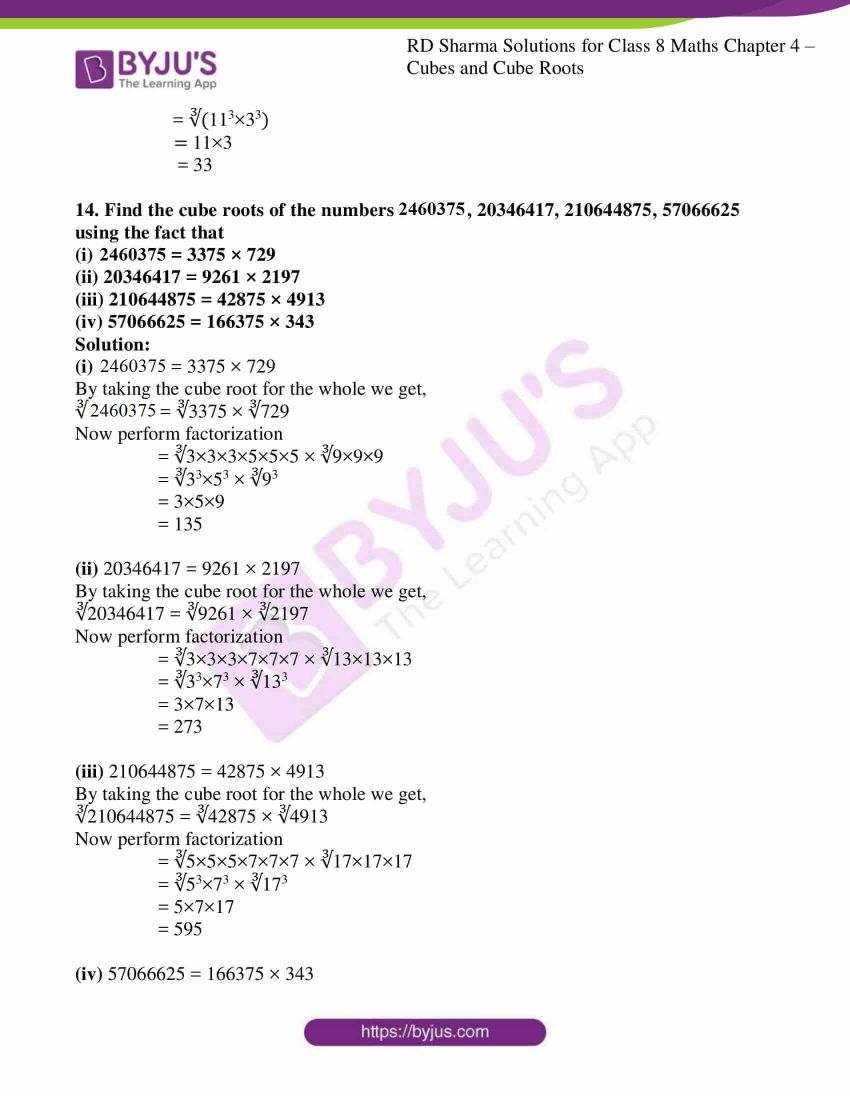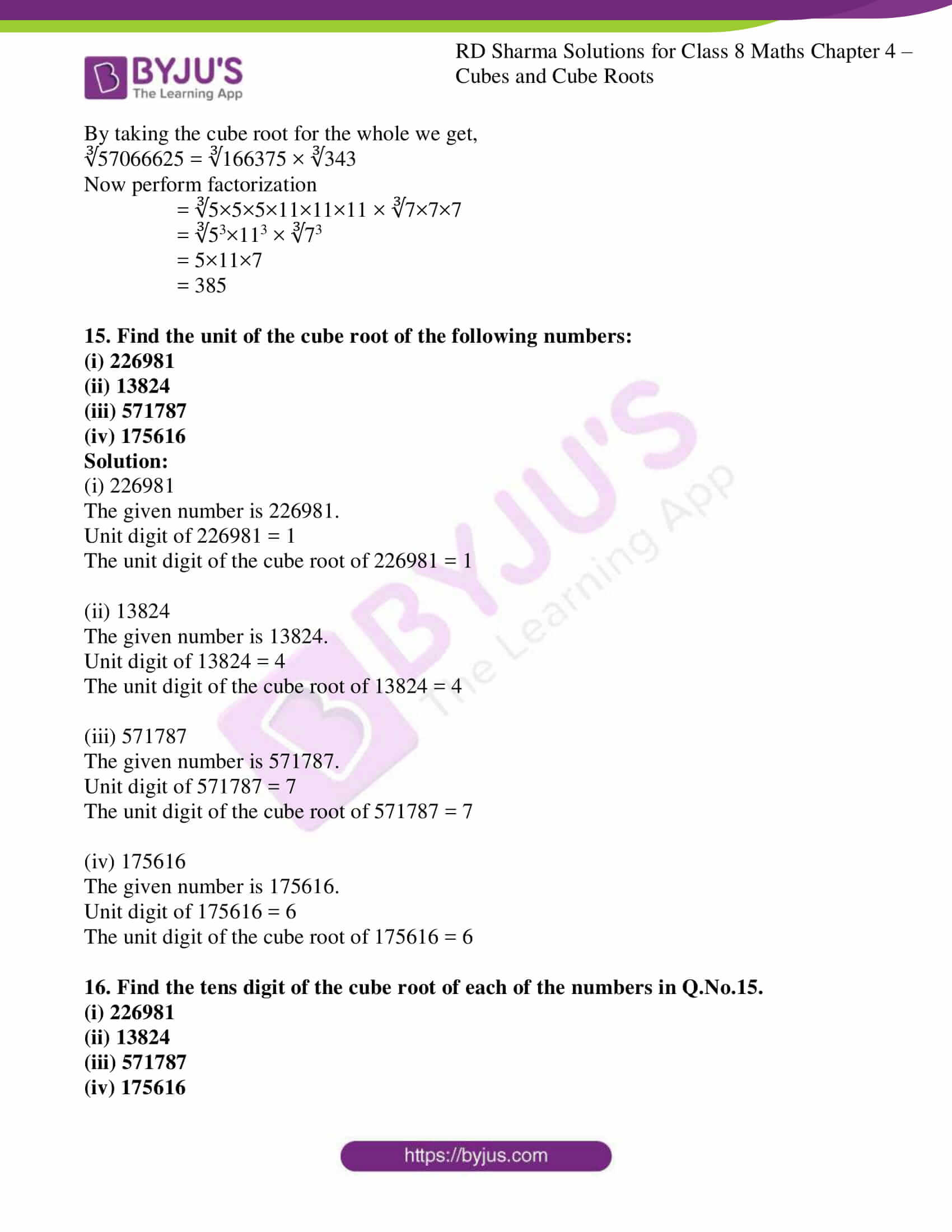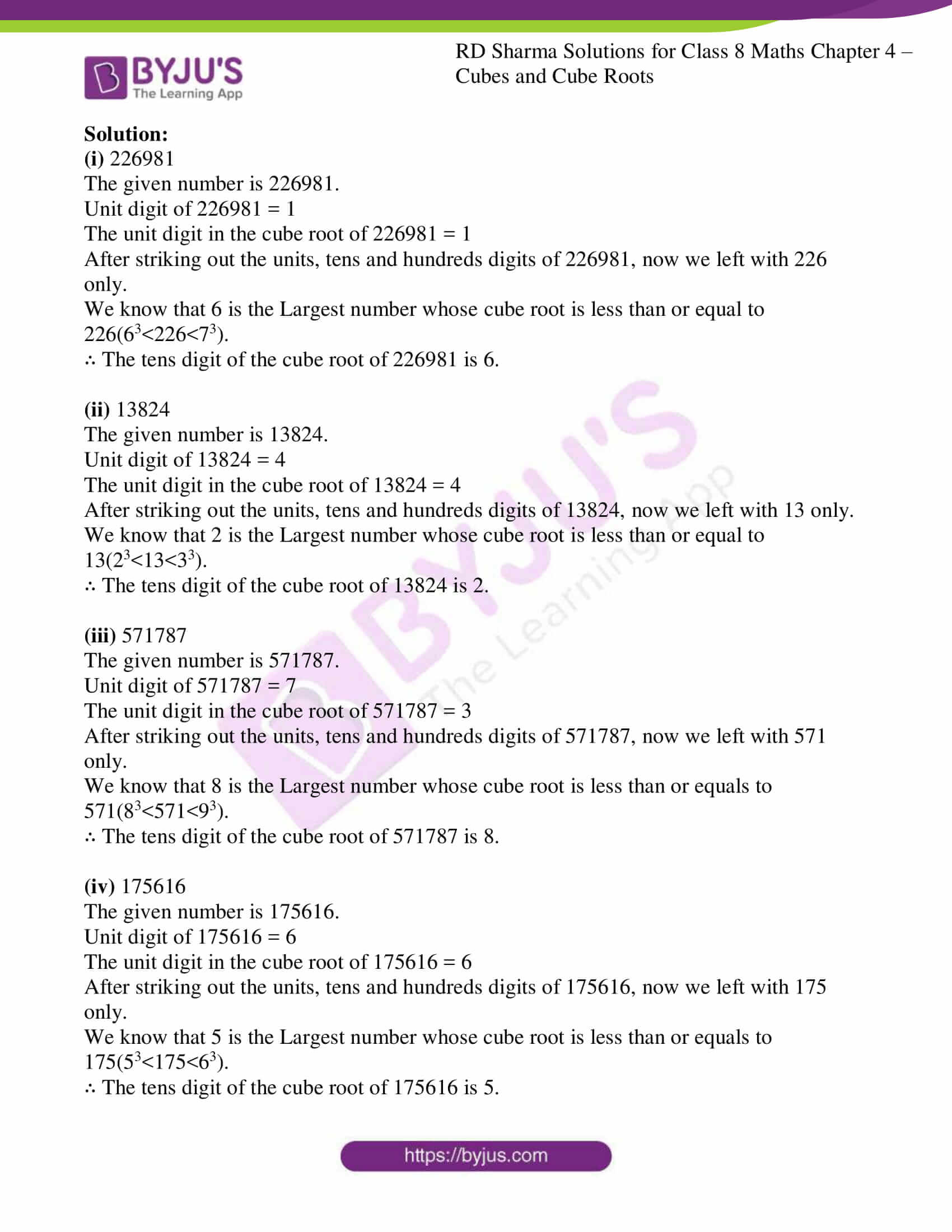### Access Answers to RD Sharma Solutions for Class 8 Maths Exercise 4.4 Chapter 4 Cubes and Cube Roots

1. Find the cube roots of each of the following integers:
(i)-125 (ii) -5832
(iii)-2744000 (iv) -753571
(v) -32768

Solution:

(i) -125

The cube root of -125 is

-125 = ∛-125 = -∛125 = -∛ (5×5×5) = -5

(ii) -5832

The cube root of -5832 is

-5832 = ∛-5832 = -∛5832

To find the cube root of 5832, we shall use the method of unit digits.

Let us consider the number 5832. Where, unit digit of 5832 = 2

Unit digit in the cube root of 5832 will be 8

After striking out the units, tens and hundreds digits of 5832,

Now we left with 5 only.

We know that 1 is the Largest number whose cube is less than or equal to 5.

So, the tens digit of the cube root of 5832 is 1.

∛-5832 = -∛5832 = -18

(iii) -2744000

∛-2744000 = -∛2744000

We shall use the method of factorization to find the cube root of 2744000

So let’s find the prime factors for 2744000

2744000 = 2×2×2×2×2×2×5×5×5×7×7×7

Now by grouping the factors into triples of equal factors, we get,

2744000 = (2×2×2) × (2×2×2) × (5×5×5) × (7×7×7)

Since all the prime factors of 2744000 is grouped in to triples of equal factors and no factor is left over.

So now take one factor from each group and by multiplying we get,

2×2×5×7 = 140

Thereby we can say that 2744000 is a cube of 140

∴ ∛-2744000 = -∛2744000 = -140

(iv) -753571

∛-753571 = -∛753571

We shall use the unit digit method,

Let us consider the number 753571, where unit digit = 1

Unit digit in the cube root of 753571 will be 1

After striking out the units, tens and hundreds digits of 753571,

Now we left with 753.

We know that 9 is the Largest number whose cube is less than or equal to 753(93<753<103).

So, the tens digit of the cube root of 753571 is 9.

∛753571 = 91

∛-753571 = -∛753571 = -91

(v) -32768

∛-32765 = -∛32768

We shall use the unit digit method,

Let us consider the Number = 32768, where unit digit = 8

Unit digit in the cube root of 32768 will be 2

After striking out the units, tens and hundreds digits of 32768,

Now we left with 32.

As we know that 9 is the Largest number whose cube is less than or equals to 32(33<32<43).

So, the tens digit of the cube root of 32768 is 3.

∛32765 = 32

∛-32765 = -∛32768 = -32

2. Show that:

(i) ∛27 × ∛64 = ∛ (27×64)

(ii) ∛ (64×729) = ∛64 × ∛729

(iii) ∛ (-125×216) = ∛-125 × ∛216

(iv) ∛ (-125×-1000) = ∛-125 × ∛-1000

Solution:

(i) ∛27 × ∛64 = ∛ (27×64)

Let us consider LHS ∛27 × ∛64

∛27 × ∛64 = ∛(3×3×3) × ∛(4×4×4)

= 3×4

= 12

Let us consider RHS ∛ (27×64)

∛ (27×64) = ∛ (3×3×3×4×4×4)

= 3×4

= 12

∴ LHS = RHS, the given equation is verified.

(ii) ∛ (64×729) = ∛64 × ∛729

Let us consider LHS ∛ (64×729)

∛ (64×729) = ∛ (4×4×4×9×9×9)

= 4×9

= 36

Let us consider RHS ∛64 × ∛729

∛64 × ∛729 = ∛(4×4×4) × ∛(9×9×9)

= 4×9

= 36

∴ LHS = RHS, the given equation is verified.

(iii) ∛ (-125×216) = ∛-125 × ∛216

Let us consider LHS ∛ (-125×216)

∛ (-125×216) = ∛ (-5×-5×-5×2×2×2×3×3×3)

= -5×2×3

= -30

Let us consider RHS ∛-125 × ∛216

∛-125 × ∛216 = ∛(-5×-5×-5) × ∛(2×2×2×3×3×3)

= -5×2×3

= -30

∴ LHS = RHS, the given equation is verified.

(iv) ∛ (-125×-1000) = ∛-125 × ∛-1000

Let us consider LHS ∛ (-125×-1000)

∛ (-125×-1000) = ∛ (-5×-5×-5×-10×-10×-10)

= -5×-10

= 50

Let us consider RHS ∛-125 × ∛-1000

∛-125 × ∛-1000 = ∛(-5×-5×-5) × ∛(-10×-10×-10)

= -5×-10

= 50

∴ LHS = RHS, the given equation is verified.

3. Find the cube root of each of the following numbers:
(i) 8×125

(ii) -1728×216
(iii) -27×2744

(iv) -729×-15625

Solution:

(i) 8×125

We know that for any two integers a and b, ∛ (a×b) = ∛a × ∛b

By using the property

∛ (8×125) = ∛8 × ∛125

= ∛ (2×2×2) × ∛ (5×5×5)

= 2×5

= 10

(ii) -1728×216

We know that for any two integers a and b, ∛ (a×b) = ∛a × ∛b

By using the property

∛ (-1728×216) = ∛-1728 × ∛216

We shall use the unit digit method

Let us consider the number 1728, where Unit digit = 8

The unit digit in the cube root of 1728 will be 2

After striking out the units, tens and hundreds digits of the given number, we are left with the 1.

We know 1 is the largest number whose cube is less than or equal to 1.

So, the tens digit of the cube root of 1728 = 1

∛1728 = 12

Now, let’s find the prime factors for 216 = 2×2×2×3×3×3

By grouping the factors in triples of equal factor, we get,

216 = (2×2×2) × (3×3×3)

By taking one factor from each group we get,

∛216 = 2×3 = 6

∴ by equating the values in the given equation we get,

∛ (-1728×216) = ∛-1728 × ∛216

= -12 × 6

= -72

(iii) -27×2744

We know that for any two integers a and b, ∛ (a×b) = ∛a × ∛b

By using the property

∛ (-27×2744) = ∛-27 × ∛2744

We shall use the unit digit method

Let us consider the number 2744, where Unit digit = 4

The unit digit in the cube root of 2744 will be 4

After striking out the units, tens and hundreds digits of the given number, we are left with the 2.

We know 2 is the largest number whose cube is less than or equal to 2.

So, the tens digit of the cube root of 2744 = 2

∛2744 = 14

Now, let’s find the prime factors for 27 = 3×3×3

By grouping the factors in triples of equal factor, we get,

27 = (3×3×3)

Cube root of 27 is

∛27 = 3

∴ by equating the values in the given equation we get,

∛ (-27×2744) = ∛-27 × ∛2744

= -3 × 14

= -42

(iv) -729×-15625

We know that for any two integers a and b, ∛ (a×b) = ∛a × ∛b

By using the property

∛ (-729×-15625) = ∛-729 × ∛-15625

We shall use the unit digit method

Let us consider the number 15625, where Unit digit = 5

The unit digit in the cube root of 15625 will be 5

After striking out the units, tens and hundreds digits of the given number, we are left with the 15.

We know 15 is the largest number whose cube is less than or equal to 15(23<15<33).

So, the tens digit of the cube root of 15625 = 2

∛15625 = 25

Now, let’s find the prime factors for 729 = 9×9×9

By grouping the factors in triples of equal factor, we get,

729 = (9×9×9)

Cube root of 729 is

∛729 = 9

∴ by equating the values in the given equation we get,

∛ (-729×-15625) = ∛-729 × ∛-15625

= -9 × -25

= 225

4. Evaluate:

(i) ∛ (43 × 63)

(ii) ∛ (8×17×17×17)

(iii) ∛ (700×2×49×5)

(iv) 125 ∛a6 – ∛125a6

Solution:

(i) ∛ (43 × 63)

We know that for any two integers a and b, ∛ (a×b) = ∛a × ∛b

By using the property

∛ (43 × 63) = ∛43 × ∛63

= 4 × 6

= 24

(ii) ∛ (8×17×17×17)

We know that for any two integers a and b, ∛ (a×b) = ∛a × ∛b

By using the property

∛ (8×17×17×17) = ∛8 × ∛17×17×17

= ∛23 × ∛173

= 2 × 17

= 34

(iii) ∛ (700×2×49×5)

Firstly let us find the prime factors for the above numbers

∛ (700×2×49×5) = ∛ (2×2×5×5×7×2×7×7×5)

= ∛ (23×53×73)

= 2×5×7

= 70

(iv) 125 ∛a6 – ∛125a6

125 ∛a6 – ∛125a6 = 125 ∛(a2)3 – ∛53(a2)3

= 125a2 – 5a2

= 120a2

5. Find the cube root of each of the following rational numbers:

(i) -125/729

(ii) 10648/12167

(iii) -19683/24389

(iv) 686/-3456

(v) -39304/-42875

Solution:

(i) -125/729

Let us find the prime factors of 125 and 729

-125/729 = – (∛ (5×5×5)) / (∛ (9×9×9))

= – (∛ (53)) / (∛ (93))

= – 5/9

(ii) 10648/12167

Let us find the prime factors of 10648 and 12167

10648/12167 = (∛ (2×2×2×11×11×11)) / (∛ (23×23×23))

= (∛ (23×113)) / (∛ (233))

= (2×11)/23

= 22/23

(iii) -19683/24389

Let us find the prime factors of 19683 and 24389

-19683/24389 = -(∛ (3×3×3×3×3×3×3×3×3)) / (∛ (29×29×29))

= – (∛ (33×33×33)) / (∛ (293))

= – (3×3×3)/29

= – 27/29

(iv) 686/-3456

Let us find the prime factors of 686 and -3456

686/-3456 = = -(∛ (2×7×7×7)) / (∛ (27×33))

= – (∛ (2×73)) / (∛ (27×33))

= – (∛ (73)) / (∛ (26×33))

= – 7/(2×2×3)

= – 7/12

(v) -39304/-42875

Let us find the prime factors of -39304 and -42875

-39304/-42875 = -(∛ (2×2×2×17×17×17)) / -(∛ (5×5×5×7×7×7))

= – (∛ (23×173)) / -(∛ (53×73))

= – (2×17)/-( 5×7)

= – 34/-35

= 34/35

6. Find the cube root of each of the following rational numbers:
(i) 0.001728
(ii) 0.003375
(iii) 0.001
(iv) 1.331

Solution:

(i) 0.001728

0.001728 = 1728/1000000

∛ (0.001728) = ∛1728 / ∛1000000

Let us find the prime factors of 1728 and 1000000

∛(0.001728) = ∛(23×23×33) / ∛(1003)

= (2×2×3)/100

= 12/100

= 0.12

(ii) 0.003375

0.003375 = 3375/1000000

∛ (0.003375) = ∛3375 / ∛1000000

Let us find the prime factors of 3375 and 1000000

∛(0.003375) = ∛(33×53) / ∛(1003)

= (3×5)/100

= 15/100

= 0.15

(iii) 0.001

0.001 = 1/1000

∛ (0.001) = ∛1 / ∛1000

= 1/ ∛103

= 1/10

= 0.1

(iv) 1.331

1.331 = 1331/1000

∛ (1.331) = ∛1331 / ∛1000

Let us find the prime factors of 1331 and 1000

∛(1.331) = ∛(113) / ∛(103)

= 11/10

= 1.1

7. Evaluate each of the following:

(i) ∛27 + ∛0.008 + ∛0.064

(ii) ∛1000 + ∛0.008 – ∛0.125

(iii) ∛(729/216) × 6/9

(iv) ∛(0.027/0.008) ÷ ∛(0.09/0.04) – 1
(v) ∛(0.1×0.1×0.1×13×13×13)

Solution:

(i) ∛27 + ∛0.008 + ∛0.064

Let us simplify

∛ (3×3×3) + ∛ (0.2×0.2×0.2) + ∛ (0.4×0.4×0.4)

∛ (3)3 + ∛ (0.2)3 + ∛ (0.4)3

3 + 0.2 + 0.4

3.6

(ii) ∛1000 + ∛0.008 – ∛0.125

Let us simplify

∛ (10×10×10) + ∛ (0.2×0.2×0.2) – ∛ (0.5×0.5×0.5)

∛ (10)3 + ∛ (0.2)3 – ∛ (0.5)3

10 + 0.2 – 0.5

9.7

(iii) ∛ (729/216) × 6/9

Let us simplify

∛ (9×9×9/6×6×6) × 6/9

(∛ (9)3 / ∛ (6)3)× 6/9

9/6 × 6/9

1

(iv) ∛(0.027/0.008) ÷ √(0.09/0.04) – 1

Let us simplify ∛(0.027/0.008) ÷ √ (0.09/0.04)

∛(0.3×0.3×0.3/0.2×0.2×0.2) ÷ √(0.3×0.3/0.2×0.2)

(∛(0.3)3 / ∛(0.2)3) ÷ (√(0.3)2 / √(0.2)2)

(0.3/0.2) ÷ (0.3/0.2) -1

(0.3/0.2 × 0.2/0.3) -1

1 – 1

0

(v) ∛(0.1×0.1×0.1×13×13×13)

∛(0.13×133)

0.1 × 13 = 1.3

8. Show that:

(i) ∛ (729)/ ∛ (1000) = ∛ (729/1000)

(ii) ∛ (-512)/ ∛ (343) = ∛ (-512/343)

Solution:

(i) ∛ (729)/ ∛ (1000) = ∛ (729/1000)

Let us consider LHS ∛ (729)/ ∛ (1000)

∛ (729)/ ∛ (1000) = ∛ (9×9×9)/ ∛ (10×10×10)

= ∛ (93/103)

= 9/10

Let us consider RHS ∛ (729/1000)

∛ (729/1000) = ∛ (9×9×9/10×10×10)

= ∛ (93/103)

= 9/10

∴ LHS = RHS

(ii) ∛ (-512)/ ∛ (343) = ∛ (-512/343)

Let us consider LHS ∛ (-512)/ ∛ (343)

∛ (-512)/ ∛ (343) = ∛-(8×8×8)/ ∛ (7×7×7)

= ∛-(83/73)

= -8/7

Let us consider RHS ∛ (-512/343)

∛ (-512/343) = = ∛-(8×8×8/7×7×7)

= ∛-(83/73)

= -8/7

∴ LHS = RHS

9. Fill in the blanks:

(i) ∛(125×27) = 3 × …

(ii) ∛(8×…) = 8
(iii) ∛1728 = 4 × …

(iv) ∛480 = ∛3×2× ∛..

(v) ∛… = ∛7 × ∛8

(vi) ∛..= ∛4 × ∛5 × ∛6

(vii) ∛(27/125) = …/5

(viii) ∛(729/1331) = 9/…

(ix) ∛(512/…) = 8/13

Solution:

(i) ∛(125×27) = 3 × …

Let us consider LHS ∛(125×27)

∛(125×27) = ∛(5×5×5×3×3×3)

= ∛(53×33)

= 5×3 or 3×5

(ii) ∛(8×…) = 8

Let us consider LHS ∛(8×…)

∛(8×8×8) = ∛83 = 8

(iii) ∛1728 = 4 × …

Let us consider LHS

∛1728 = ∛(2×2×2×2×2×2×3×3×3)

= ∛(23×23×33)

= 2×2×3

= 4×3

(iv) ∛480 = ∛3×2× ∛..

Let us consider LHS

∛480 = ∛(2×2×2×2×2×3×5)

= ∛(23×22×3×5)

= ∛23× ∛3 × ∛2×2×5

= 2 × ∛3 × ∛20

(v) ∛… = ∛7 × ∛8

Let us consider RHS

∛7 × ∛8 = ∛(7 × 8)

= ∛56

(vi) ∛..= ∛4 × ∛5 × ∛6

Let us consider RHS

∛4 × ∛5 × ∛6 = ∛(4 × 5 × 6)

= ∛120

(vii) ∛(27/125) = …/5

Let us consider LHS

∛(27/125) = ∛(3×3×3)/(5×5×5)

= ∛(33)/(53)

= 3/5

(viii) ∛(729/1331) = 9/…

Let us consider LHS

∛(729/1331) = ∛(9×9×9)/(11×11×11)

= ∛(93)/(113)

= 9/11

(ix) ∛(512/…) = 8/13

Let us consider LHS

∛(512/…) = ∛(2×2×2×2×2×2×2×2×2)

= ∛(23×23×23)

= 2×2×2

= 8

So, 8/∛… = 8/13

when numerators are same the denominators will also become equal.

8 × 13 = 8 × ∛…

∛…= 13

… = (13)3

= 2197

10. The volume of a cubical box is 474. 552 cubic metres. Find the length of each side of the box.

Solution:

Volume of a cubical box is 474.552 cubic metres

V = 83,

Let ‘S’ be the side of the cube

83 = 474.552 cubic metres

8 = ∛474.552

= ∛ (474552/1000)

Let us factorise 474552 into prime factors, we get:

474552 = 2×2×2×3×3×3×13×13×13

By grouping the factors in triples of equal factors, we get:

474552 = (2×2×2) × (3×3×3) × (13×13×13)

Now, ∛474.552 = ∛ ((2×2×2) × (3×3×3) × (13×13×13))

= 2×3×13

= 78

Also,

∛1000 = ∛ (10×10×10)

= ∛ (10)3

= 10

So now let us equate in the above equation we get,

8 = ∛ (474552/1000)

= 78/10

= 7.8

∴ length of the side is 7.8m.

11. Three numbers are to one another 2:3:4. The sum of their cubes is 0.334125. Find the numbers.

Solution:

Let us consider the ratio 2:3:4 be 2a, 3a, and 4a.

So according to the question:

(2a)3+ (3a)3+(4a)3 = 0.334125

8a3+27 a3+64 a3 = 0.334125

99a3 = 0.334125

a3 = 334125/1000000×99

= 3375/1000000

a = ∛ (3375/1000000)

= ∛((15×15×15)/ 100×100×100)

= 15/100

= 0.15

∴ The numbers are:

2×0.15 = 0.30

3×0.15 = 0.45

4×0.15 = 0.6

12. Find the side of a cube whose volume is 24389/216m3.

Solution:

Volume of the side s = 24389/216 = v

V= 8.3

8 = ∛v

= ∛(24389/216)

By performing factorisation we get,

= ∛(29×29×29/2×2×2×3×3×3)

= 29/(2×3)

= 29/6

∴ The length of the side is 29/6.

13. Evaluate:

(i) ∛36 × ∛384

(ii) ∛96 × ∛144

(iii) ∛100 × ∛270

(iv) ∛121 × ∛297

Solution:

(i) ∛36 × ∛384

We know that ∛a × ∛b = ∛ (a×b)

By using the above formula let us simplify

∛36 × ∛384 = ∛ (36×384)

The prime factors of 36 and 384 are

= ∛ (2×2×3×3) × (2×2×2×2×2×2×3×3×3)

= ∛(23×23×23×33)

= 2×2×2×3

= 24

(ii) ∛96 × ∛144

We know that ∛a × ∛b = ∛ (a×b)

By using the above formula let us simplify

∛96 × ∛144 = ∛ (96×144)

The prime factors of 96 and 144 are

= ∛ (2×2×2×2×2×3) × (2×2×2×2×3×3)

= ∛(23×23×23×33)

= 2×2×2×3

= 24

(iii) ∛100 × ∛270

We know that ∛a × ∛b = ∛ (a×b)

By using the above formula let us simplify

∛100 × ∛270 = ∛ (100×270)

The prime factors of 100 and 270 are

= ∛ (2×2×5×5) × (2×3×3×3×5)

= ∛(23×33×53)

= 2×3×5

= 30

(iv) ∛121 × ∛297

We know that ∛a × ∛b = ∛ (a×b)

By using the above formula let us simplify

∛121 × ∛297 = ∛ (121×297)

The prime factors of 121 and 297 are

= ∛ (11×11) × (3×3×3×11)

= ∛(113×33)

= 11×3

= 33

14. Find the cube roots of the numbers 2460375, 20346417, 210644875, 57066625 using the fact that
(i) 2460375 = 3375 × 729
(ii) 20346417 = 9261 × 2197
(iii) 210644875 = 42875 × 4913
(iv) 57066625 = 166375 × 343

Solution:

(i) 2460375 = 3375 × 729

By taking the cube root for the whole we get,

∛2460375 = ∛3375 × ∛729

Now perform factorization

= ∛3×3×3×5×5×5 × ∛9×9×9

= ∛33×53 × ∛93

= 3×5×9

= 135

(ii) 20346417 = 9261 × 2197

By taking the cube root for the whole we get,

∛20346417 = ∛9261 × ∛2197

Now perform factorization

= ∛3×3×3×7×7×7 × ∛13×13×13

= ∛33×73 × ∛133

= 3×7×13

= 273

(iii) 210644875 = 42875 × 4913

By taking the cube root for the whole we get,

∛210644875 = ∛42875 × ∛4913

Now perform factorization

= ∛5×5×5×7×7×7 × ∛17×17×17

= ∛53×73 × ∛173

= 5×7×17

= 595

(iv) 57066625 = 166375 × 343

By taking the cube root for the whole we get,

∛57066625 = ∛166375 × ∛343

Now perform factorization

= ∛5×5×5×11×11×11 × ∛7×7×7

= ∛53×113 × ∛73

= 5×11×7

= 385

15. Find the unit of the cube
root of the following numbers:
(i) 226981
(ii) 13824
(iii) 571787
(iv) 175616

Solution:

(i) 226981

The given number is 226981.

Unit digit of 226981 = 1

The unit digit of the cube root of 226981 = 1

(ii) 13824

The given number is 13824.

Unit digit of 13824 = 4

The unit digit of the cube root of 13824 = 4

(iii) 571787

The given number is 571787.

Unit digit of 571787 = 7

The unit digit of the cube root of 571787 = 7

(iv) 175616

The given number is 175616.

Unit digit of 175616 = 6

The unit digit of the cube root of 175616 = 6

16. Find the tens digit of the cube root of each of the numbers in Q.No.15.
(i) 226981
(ii) 13824
(iii) 571787
(iv) 175616

Solution:

(i) 226981

The given number is 226981.

Unit digit of 226981 = 1

The unit digit in the cube root of 226981 = 1

After striking out the units, tens and hundreds digits of 226981, now we left with 226 only.

We know that 6 is the Largest number whose cube root is less than or equal to 226(63<226<73).

∴ The tens digit of the cube root of 226981 is 6.

(ii) 13824

The given number is 13824.

Unit digit of 13824 = 4

The unit digit in the cube root of 13824 = 4

After striking out the units, tens and hundreds digits of 13824, now we left with 13 only.

We know that 2 is the Largest number whose cube root is less than or equal to 13(23<13<33).

∴ The tens digit of the cube root of 13824 is 2.

(iii) 571787

The given number is 571787.

Unit digit of 571787 = 7

The unit digit in the cube root of 571787 = 3

After striking out the units, tens and hundreds digits of 571787, now we left with 571 only.

We know that 8 is the Largest number whose cube root is less than or equals to 571(83<571<93).

∴ The tens digit of the cube root of 571787 is 8.

(iv) 175616

The given number is 175616.

Unit digit of 175616 = 6

The unit digit in the cube root of 175616 = 6

After striking out the units, tens and hundreds digits of 175616, now we left with 175 only.

We know that 5 is the Largest number whose cube root is less than or equals to 175(53<175<63).

∴ The tens digit of the cube root of 175616 is 5.